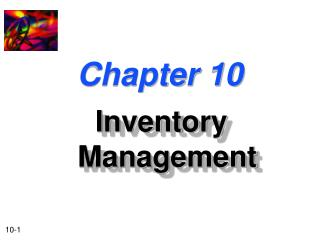DownloadDownload PresentationChapter 10

# Chapter 10

Télécharger la présentation## Chapter 10

- - - - - - - - - - - - - - - - - - - - - - - - - - - E N D - - - - - - - - - - - - - - - - - - - - - - - - - - -
##### Presentation Transcript

1. Chapter 10 Inventory Management

2. Inventory Management and Control • Inventories form a link between production and sale of a product. • Inventory types: • Raw-materials inventory • In-transit inventory • Finished-goods inventories

3. Inventory Management and Control • Purchasing • Production scheduling • Efficient servicing of customer demands • Inventories provide flexibility for the firm in:

4. Appropriate Level of Inventories How does a firm determine the appropriate level of inventories? Employ a cost-benefit analysis. Compare the benefits of economies of production, purchasing, and product marketing against the cost of the additional investment in inventories.

5. ABC Method of Inventory Control ABC method of inventory control Method which controls expensive inventory items more closely than less expensive items. • Review “A” items most frequently • Review “B” and “C” items less rigorously and/or less frequently. 100 90 C B 70 Cumulative Percentage of Inventory Value A 0 15 45 100 Cumulative Percentage of Items in Inventory

6. How Much to Order? • The optimal quantity to order depends on: • Forecast usage • Ordering cost • Carrying cost Ordering can mean either the purchase or production of the item.

7. Total Inventory Costs • Total inventory costs (T) = • C (Q / 2) + O (S / Q) C: Carrying costs per unit per period O: Ordering costs per order S: Total usage during the period Q Average Inventory INVENTORY (in units) Q / 2 TIME

8. Economic Order Quantity The quantity of an inventory item to order so that total inventory costs are minimized over the firm’s planning period. The EOQ or optimal quantity (Q*) is: 2 (O) (S) Q* = C

9. Example of the Economic Order Quantity Basket Wonders is attempting to determine the economic order quantity for fabric used in the production of baskets. • 10,000 yards of fabric were used at a constant rate last period. • Each order represents an ordering cost of \$200. • Carrying costs are \$1 per yard over the 100-day planning period. What is the economic order quantity?

10. Economic Order Quantity We will solve for the economic order quantity given that ordering costs are \$200 per order, total usage over the period was 10,000 units, and carrying costs are \$1 per yard (unit). 2 (\$200) (10,000) Q* = \$1 Q* = 2,000 Units

11. Total Inventory Costs • EOQ (Q*) represents the minimum point in total inventory costs. Total inventory costs Total Carrying Costs Costs Total Ordering Costs Q* Order Size (Q)

12. When to Order? • Issues to consider: • Lead Time-- The length of time between the placement of an order for an inventory item and when the item is received in inventory. Order Point -- The quantity to which inventory must fall in order to signal that an order must be placed to replenish an item. Order Point (OP) = Lead time X Daily usage

13. Example of When to Order Julie Miller of Basket Wonders has determined that it takes only 2 days to receive the order of fabric after the placement of the order. When should Julie order more fabric? Lead time = 2 days Daily usage = 10,000 yards / 100 days = 100 yards per day Order Point= 2 days x100 yards per day =200 yards

14. Example of When to Order Economic Order Quantity (Q*) 2000 UNITS Order Point 200 0 18 20 38 40 Lead Time DAYS

15. Safety Stock • Safety Stock -- Inventory stock held in reserve as a cushion against uncertain demand (or usage) and replenishment lead time. Our previous example assumed certain demand and lead time. When demand and/or lead time are uncertain, then the order point is: Order Point = (Avg. lead time x Avg. daily usage) + Safety stock

16. Order Point with Safety Stock 2200 2000 Order Point UNITS 400 200 Safety Stock 0 18 20 38 DAYS

17. Order Point with Safety Stock 2200 2000 Actual lead time is 3 days! (at day 21) The firm “dips” into the safety stock Order Point UNITS 400 200 Safety Stock 0 18 21 DAYS

18. How Much Safety Stock? • What is the proper amount amount of safety stock? • Depends on the: • Amount of uncertainty in inventory demand • Amount of uncertainty in the lead time • Cost of running out of inventory • Cost of carrying inventory

19. Just-in-Time • Just-in-Time -- An approach to inventory management and control in which inventories are acquired and inserted in production at the exact times they are needed. • Requirements of applying this approach: • A very accurate production and inventory information system • Highly efficient purchasing • Reliable suppliers • Efficient inventory-handling system